# Radial Equation Separation

Substituting the form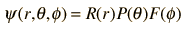into the hydrogen Schrodinger equation allows all the partial derivatives to be expressed as ordinary derivatives. Direct substitution and a bit of rearranging gives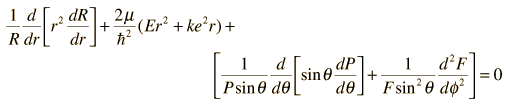Note that the first two terms contain only variable r, and the remaining terms contain the angular variables. Since the equation must hold for all values of these variables, the collection of radial terms must be equal to a constant. This separates out the radial equation. The sum of the angular terms must therefore be equal to the negative of that constant. Also, the terms in the two angles must separately equal a constant since they must hold for all angle values. This separates the two angular parts into the colatitude and azimuthal equations.

Index

Schrodinger equation concepts

Hydrogen concepts
HyperPhysics***** Quantum Physics Go Back

# The Radial Equation

Upon separation of the Schrodinger equation for the hydrogen atom, the radial equation is: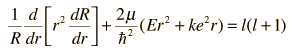In order to separate the equations, the radial part is set equal to a constant, and the form of the constant on the right above reflects the nature of the solution of the colatitude equation which yields the orbital quantum number.

Solution of these equations under the constraints placed on the wavefunction leads to series solutions in the form of polynomials called the associated Laguerre functions. In order to fit the physical boundary conditions, these solutions contain a parameter n which can take only positive integer values; this parameter is called the principal quantum number. The form of the radial solutions is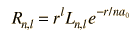where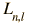is the associated Laguerre function. The first few radial wavefunctions R are shown as part of the hydrogen wavefunctions.

Index

Schrodinger equation concepts

Hydrogen concepts
HyperPhysics***** Quantum Physics Go Back

# The Principal Quantum Number

The principal quantum number or total quantum number n arises from the solution of the radial part of the Schrodinger equation for the hydrogen atom. The bound state energies of the electron in the hydrogen atom are given by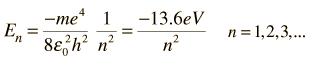In the notation of the periodic table, the main shells of electrons are labled K(n=1),L(n=2),M(n=3), etc. based on the principal quantum number.

 Quantum numbers for atomic electrons
Index

Schrodinger equation concepts

Hydrogen concepts
HyperPhysics***** Quantum Physics Go Back